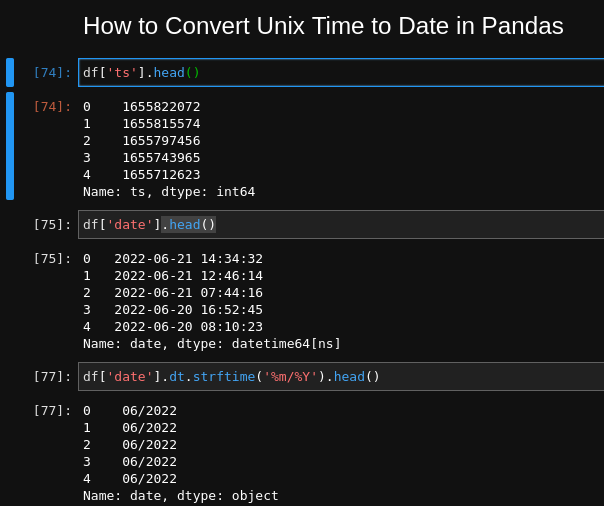To convert Unix timestamp to readable date in Pandas we can use method: `pd.to_datetime`

``````df['date'] = pd.to_datetime(df['date'],unit='s')
``````

So this will convert:

``````[1655822072.437469, 1655815574.333629, 1655797456.516109]
``````

to datetime in Pandas:

``````DatetimeIndex(['2022-06-21 14:34:32.437469006',
'2022-06-21 12:46:14.333628893',
'2022-06-21 07:44:16.516108990'],
dtype='datetime64[ns]', freq=None)
``````

Let's cover all the steps in to practical example - converting Unix timestamp to any date format (including dd/mm/yyyy).

## Setup

Suppose we have DataFrame with Unix timestamp column as follows:

``````dict = {'ts': {0: 1655822072.437469,
1: 1655815574.333629,
2: 1655797456.516109,
3: 1655743965.358579,
4: 1655712623.707739},
'reply_count': {0: 2.0, 1: 3.0, 2: 3.0, 3: 2.0, 4: None}}

pd.DataFrame(dict)
``````

So data will look like:

0 1655822072.437469 2.0
1 1655815574.333629 3.0
2 1655797456.516109 3.0
3 1655743965.358579 2.0
4 1655712623.707739 NaN## Step 1: Convert Unix time column to datetime

The first step is to convert the Unix timestamp to Pandas datetime by:

``````df['date'] = pd.to_datetime(df['ts'], unit='s')
``````

The important part of the conversion is `unit='s'` which stands for seconds. There other options like:

• `ns` - nanoseconds
• `ms` - milliseconds

Default value is None and all available options can be found here: pandas.Timestamp

× Pro Tip 1
Sometimes the Unix time can be stored as a string - so conversion to integer may be needed:
`.astype(int)`
``````df['ts'] = df['ts'].astype(int)
``````

## Step 2: Convert Unix time to readable date

The second step is to convert Pandas datetime to a readable date. This is possible by using `dt` attribute:

``````df['date'].dt.date
``````

The output will be the date component of the original Unit time:

``````0      2022-06-21
1      2022-06-21
2      2022-06-21
3      2022-06-20
4      2022-06-20
``````

## Step 3: Convert Unix time to readable time

To convert the Unix time to a well formatted time string we can use again the `dt` attribute:

``````df['date'].dt.time
``````

will give us:

``````0      14:34:32.437468
1      12:46:14.333628
2      07:44:16.516109
3      16:52:45.358578
4      08:10:23.707739
``````

## Step 4: Convert Unix time to custom date or time format

Suppose we would like to get different time pattern like:

• `dd/mm/yy`
• `HH:MM`
etc

This is possible by using method `.dt.strftime()`:

``````df['date'].dt.strftime('%m/%Y')
``````

which will result into:

``````0      06/2022
1      06/2022
2      06/2022
3      06/2022
4      06/2022
``````

To find more examples you can consult with: How to Extract Month and Year from DateTime column in Pandas

## Step 5: Use datetime.datetime.utcfromtimestamp

Alternative solution is to use `datetime.datetime.utcfromtimestamp` to convert Unix timestamp to date in Pandas.

To use method like `datetime.utcfromtimestamp` we will need to apply it to the Unix column:

``````from datetime import datetime
df["ts"].apply(lambda x: datetime.utcfromtimestamp(x).strftime('%Y-%m-%dT%H:%M:%SZ'))
``````

In this way we can specify the format like:

• `%Y-%m-%dT%H:%M:%SZ`
• `%d-%m-%Y %H:%M:%S`

## Conclusion

In this article, we saw multiple ways to convert timestamp columns to datetime.

We also covered multiple date and time formats, plus possible problems.